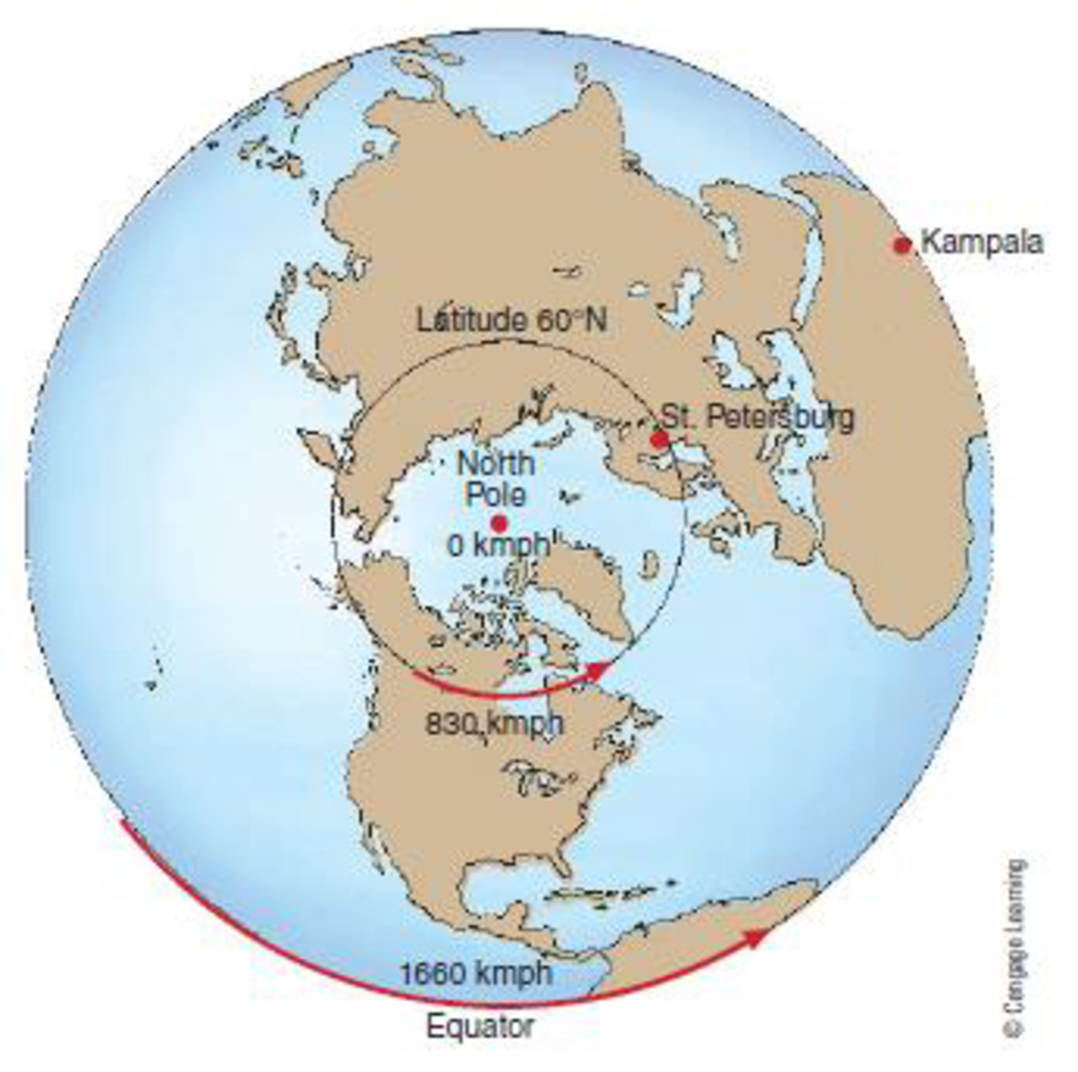Chapter 1, Problem 21FQ

Chapter
Section
Textbook Problem

How much faster does a point on the equator move than a point at 60°N latitude?To determine

The speed at which a point on the equator moves when compared to that at the 60N latitude.

Explanation

The speed of rotation on the Earth differs on account of the distance from equator. The distance covered by a point on the equator in 24 hours will be the largest and hence, the Earth possess the maximum linear velocity at the equator.

At Kampala, Uganda, located close to the equator, the velocity is approximately 1,660 kilometers per hour. When the velocity at St

Still sussing out bartleby?

Check out a sample textbook solution.

See a sample solution

The Solution to Your Study Problems

Bartleby provides explanations to thousands of textbook problems written by our experts, many with advanced degrees!

Get Started

Find more solutions based on key concepts